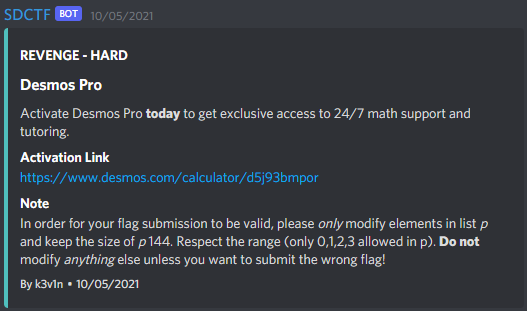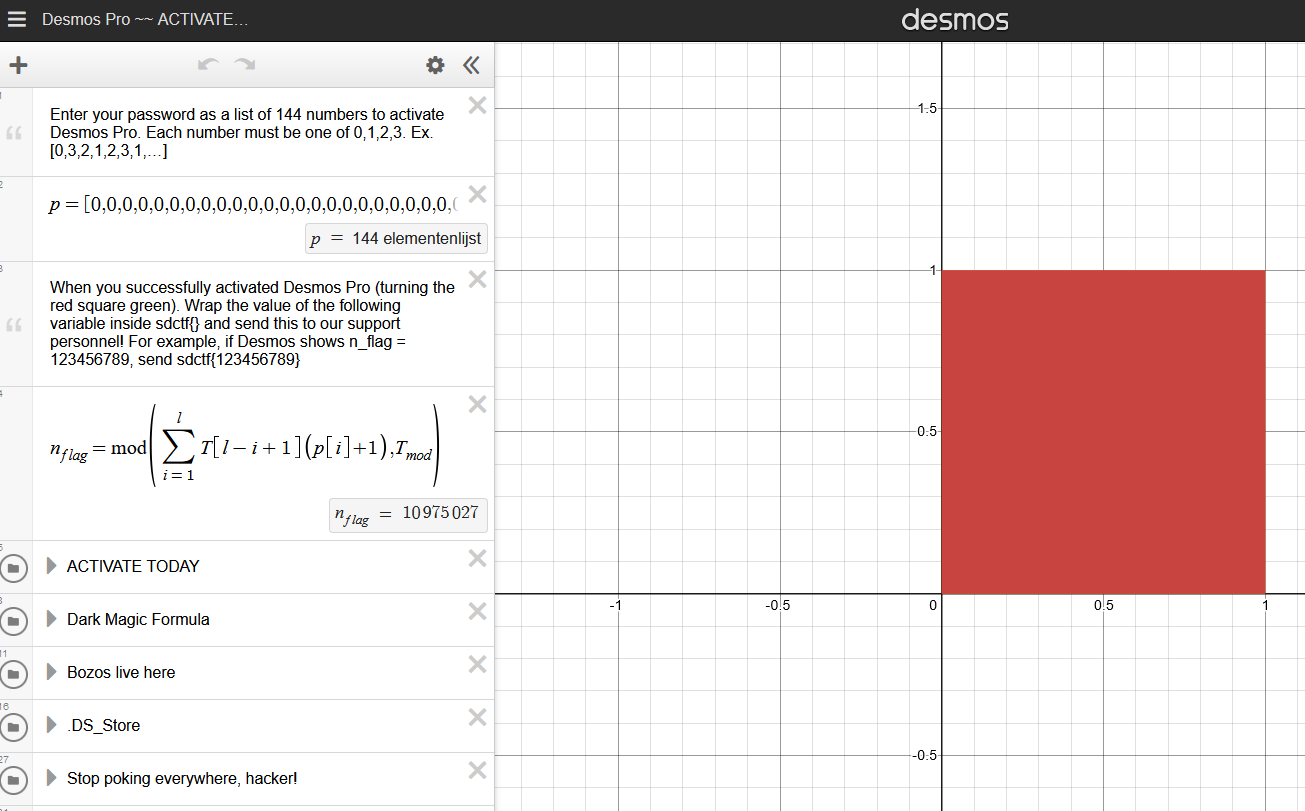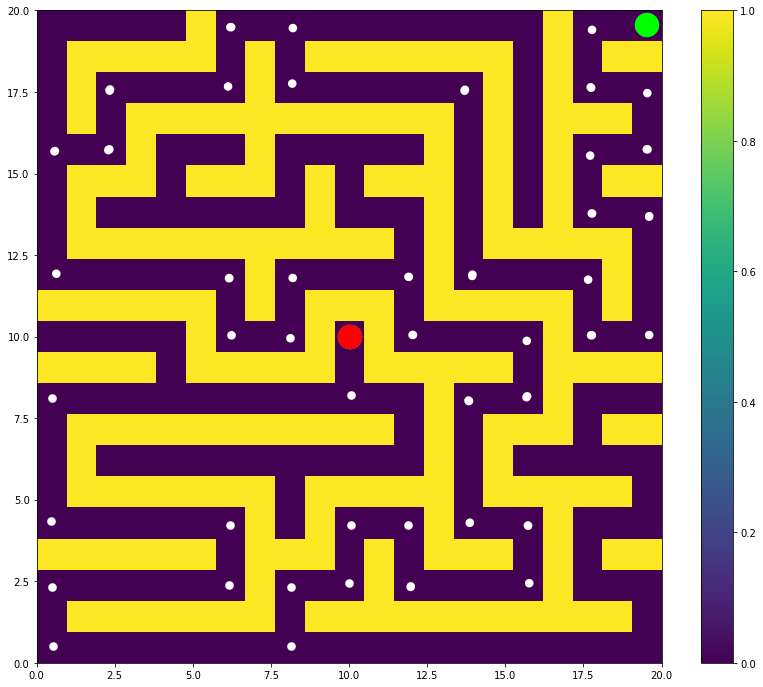# San Diego CTF 2021 - Desmos Pro

Reversing – 799 pts (5 solves) – Chall author: k3v1n

Math lock created in Desmos. Reversable through mathematical investigation.

## ChallengeWe are linked to an online Desmos file (see link above), where we are greeted by a red square. If we are able to turn the red square to green, we are able to retrieve the flag.## Solution

Upon inspection we find that in order to turn the square green we need to find the correct sequence p, consisting of 144 elements in {0,1,2,3}. Clearly not brute-forcable :c.

The criteria for the colouring of the square reveal that we need to find p such that C=0,

C=\left|X\left(l\right)-x_{f}\right|+\left|Y\left(l\right)-y_{f}\right|+\sum_{j=0}^{l}M\left(g\left(0,X\left(j\right),x_{max}-1\right),g\left(0,Y\left(j\right),y_{max}-1\right)\right)


From the above we can see that all three elements have to be zero in order for C to become zero. The first element, with the X function, implies that our p sequence needs to have 10 more 1’s than 3’s, whereas the second element, with the Y function, implies that our p sequence needs to have 10 more 2’s than 0’s. However, this on its own is still not enough to start brute-forcing our way through. The trick to this challenge is to understand the third element, the scary looking sum. For the sum to be zero, all individual call to M should return zero as it can only return 0 or 1. Interestingly, we note that M is dependent on sequential calls of X and Y, hence the order of p seems to matter too.

Knowing all of this, we can set-up a plan. Let us try to investigate the possible outcomes of M given values of X and Y. We know that it starts of with l=0, so X=0 and Y=20, and ends with l=144, so X=10 and Y=10. When we plot M as a function of both X and Y outputs we find some kind of maze!Like mentioned before, we start at X=0, Y=20 (green dot) and need to end up at X=10, Y=10 (red dot) in 144 steps. Through testing with the X and Y functions we find that the values of p correspond to moving through the maze in a certain direction following 0: right, 1: down, 2: left, 3: up. We can now find our solution sequence of p to be

[2,2,1,1,0,0,1,1,2,2,1,1,0,0,1,1,1,1,2,2,3,3,2,2,2,2,3,3,3,3,3,3,2,2,2,2,2,2,3,3,2,2,1,1,2,2,2,2,1,1,2,2,1,1,1,1,0,0,0,0,0,0,1,1,0,0,3,3,0,0,0,0,1,1,0,0,0,0,1,1,2,2,1,1,1,1,0,0,1,1,2,2,2,2,3,3,2,2,1,1,2,2,1,1,2,2,2,2,2,2,2,2,3,3,0,0,0,0,0,0,3,3,2,2,2,2,2,2,3,3,3,3,0,0,0,0,0,0,0,0,0,0,3,3]


from which we retrieve our flag to be

sdctf{440778777}


Ta-da!